# Uncertainty in Physical Measurements Module 2

Uncertainty Module 2 - UM Student Guide Oct. 17, 2017, 10:30 a.m.

## Digital Instruments

In this Module we will consider instruments with a digital readout. You will be using a digital caliper to measure the diameter of a coin.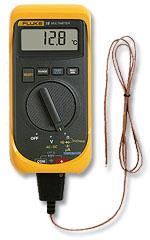The Figure shows a digital thermometer, which is reading 12.8 °C. Assume for now that the thermometer is working perfectly and the engineers who designed it did their job correctly. The value of 12.8 is called the measurand of the temperature.

##Questions

1. If the actual temperature was 12.76 °C, what would the thermometer read?

2. If the actual temperature was 12.74 °C, what would the thermometer read?

3. If the actual temperature was 12.84 °C, what would the thermometer read?

4. If the actual temperature was 12.86 °C, what would the thermometer read?

It seems clear that for a reading of 12.8 °C it is equally likely that the actual temperature is between 12.75 and 12.85 °C. Therefore if we construct a probability distribution function (pdf) similar to what we did for dice in Module 1 – Backgammon 101 it would look like Figure 1. This probability distribution is called rectangular or uniform. Note that:

• We call its height p.

• We have shaded the area between the temporarily mysterious values of $$12.80 - {a \over \sqrt{3}}$$ and ​$$12.80 + {a \over \sqrt{3}}$$​ . Soon we will clear up the mystery.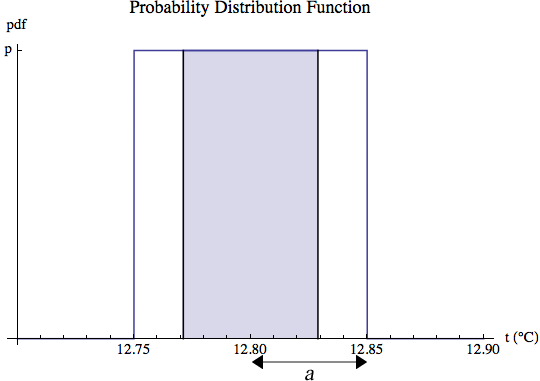#Questions

1. Just as for the triangular probability distribution you learned about it Module 1 –Backgammon 101, the total area under the probability distribution function of Figure 1 should be exactly equal to 1. What is the value of p?

2. To two significant figures what is the area under the shaded part of Figure 1?

In Module 1 we stated that it was fairly easy to show that the variance of the values for rolling dice 36 times with a result that exactly matched the theoretical prediction is equal to 6. The calculation is easy because the values that the dice can come up with are discrete integers. Suppose we have a very large number of objects whose temperatures are equally distributed between $$\bar{t}-a$$ and ​$$\bar{t}+a$$, as described by Figure 1. Now the values of the temperature can be any number between the limits, and calculating the variance requires using integrals. Using that mathematics, it can be shown than the variance of the temperatures is:

$$var={a^2 \over 3}$$        (1)

The standard deviation $$\sigma$$ is defined as the square root of the variance:

\begin{align*} \sigma & \equiv \sqrt{var} = {a \over \sqrt{3}} \\ & \cong 0.57735a \cong 0.58a \cong 0.6a \end{align*}       (2)

Note that the standard deviation is how we defined the limits of the shaded area in Figure 1. Thus, from your answer to Question 6, there is a 58% chance that the true value of the temperature, whatever it may be, is between ​$$12.80 - \sigma$$​ and ​$$12.80 + \sigma$$​ . Therefore, the standard deviation is a good measure of the standard uncertainty u of the value of the temperature: there is a 58% chance that the true value of the temperature lies within one standard deviation of the value of the measurand, i.e. of 12.8 °C.

$$u(t) = \sigma$$      (3)

For the temperature measurements the half-width a = 0.05 °C, so from Eqn. 2 the uncertainty is $$0.05 \times 0.6 = 0.03\ ^\circ {\rm C}$$. Thus we can present the value of the temperature: as:

$$t = (12.80 \pm 0.03)\ ^\circ {\rm C}$$      (4)

There is another notation that is sometimes used to express the same thing as Equation 4:

$$t = 12.80(3)\ ^\circ {\rm C}$$​    (5)

Eqns. 4 and 5 are both saying that the temperature is probably between 12.77 and 12.83 °C. Therefore, the uncertainty is telling us that there is some significance in the hundredths of a degree in the value of the measurand. So we have added an explicit zero in the hundredths place to the actual reading of 12.8. The conclusion is that in an experimental context, it is the uncertainty that defines the number of significant figures in the value of the measurand.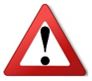You may have seen other definitions of significant figures and rules for determining how many figures are actually significant in the result of a calculation. In the context of an experimental measurement, these other definitions and rules are wrong. The uncertainty defines the number of significant figures in the result of a measurement.

What about the number of significant figures in the uncertainty itself? We will discuss this question in more detail later, but for now will just state the rule:

The number of significant figures in an experimental standard uncertainty is 1 or 2, but never greater than this.

Often we will just call the standard uncertainty just the “uncertainty” in what follows.

Occasionally an uncertainty is not symmetric about the value of the measurand. In these very rare cases one writes, for example, $$x = 1.23^{+0.05}_{-0.03}$$.At this point you may be asking yourself why we are going to all this trouble to define the standard uncertainty u as 0.6 times the half-width of the probability distribution a. Why not just define the uncertainty as the value of a? The reason is that later we will learn about probability distributions which do not have a finite half-width: the Gaussian or bell-shaped curve is a common example. So for consistency, standard uncertainties are defined the same way for all probability distributions: they are the standard deviation $$\sigma$$.

##Questions

1. Your Instructor brings a more expensive digital thermometer in, and measures the same temperature we have been considering. The readout of the thermometer is shown to the right. What is the value of the measurand and the uncertainty u in this measurement?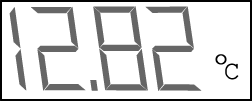2. Assume that a system has some exact temperature. What would be necessary for you to determine what this temperature is, exactly? It this possible?

3. When we write the value of a temperature and its uncertainty, we are trying to communicate to other people a range of values within which we believe the actual temperature probably lies. Assume you use the original digital thermometer which reads to a tenth of a degree, and you measure a sample to be at a temperature of 12.8 degrees C.  Numerically the uncertainty u from Eqn. 2 with a = 0.05, as read on a calculator, is 0.028 867 51. Which is these following ways of writing the value and uncertainty in the temperature best communicate the result and why?

1. $$(12.8 \pm 0.03)\ ^\circ {\rm C}$$
2. $$(12.80 \pm 0.03)\ ^\circ {\rm C}$$
3. $$(12.800 \pm 0.029)\ ^\circ {\rm C}$$
4. $$(12.8 \pm 0.029)\ ^\circ {\rm C}$$
5. $$(12.80 \pm 0.028\ 867\ 51)\ ^\circ {\rm C}$$
6. $$(12.820\ 000\ 00 \pm 0.028\ 867\ 51)\ ^\circ {\rm C}$$You may have seen the uncertainty in a measurement called the error. This is a terrible name, since “error” implies that some mistake has been made. Here there have been no mistakes but there is still an uncertainty in the measurement. So calling the uncertainty an error is an error!

The difference in the meanings of the words “uncertainty” and “error” is why, for example, it is called Heisenberg’s Uncertainty Principle, not Heisenberg’s Error Principle.

##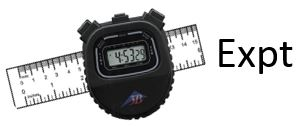Activity 1

Measure the diameter of a coin with the supplied digital caliper. Determine the uncertainty in your measurement. Write down the diameter of the coin as the measurand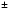the uncertainty. We will call this uncertainty the reading uncertainty uReading.

Good manufacturers of measuring instruments give the accuracy of the instrument. The manufacturer controls the tolerances of the various parts of the instrument so that the true value is withinof the accuracy. Determine the accuracy of the caliper you are using: for many of our calipers, it is printed on the back.  For the calipers supplied in MP127, there was a Technical Specifications Document in the original packaging.

It is reasonable to approximate the probability distribution function associated with the accuracy as rectangular. Make a graph of this pdf for your measurement of the diameter of the coin. Determine the uncertainty associated with this pdf. We will call this the accuracy uncertainty uAccuracy.

You now have two different uncertainties associated with your measurand of the diameter of the coin. The total uncertainty in your measurement is the combination of these two uncertainties. Since each individual uncertainty represents a 58% chance that the true value lies within $$\pm u$$ of the measurand, we want the combined uncertainty to also represent a 58% chance. It turns out that the way to combine the two uncertainties that preserves the probability is the square root of the sum of the squares of the individual uncertainties. So the total uncertainty in your measurement, uTotal, is:

$$u_{\rm Total} = \sqrt{(u_{\rm Reading})^2 + (u_{\rm Accuracy})^2}$$     (5)

This way of combining values is called quadrature.

Finally, present your final result for the diameter of the coin.

Here we will discuss why combining uncertainties using quadrature is a reasonable procedure. The discussion will not be rigorous, but is correct.

The point is that the reading uncertainty and the accuracy uncertainty are independent of each other. So when we combine them, they can cancel each other. So just adding the two values together is too pessimistic, it doesn’t allow for this possible cancellation.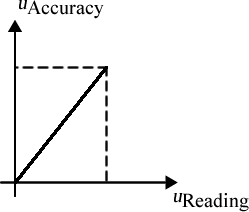If we imagine some sort of abstract error space, then the reading and accuracy uncertainties are perpendicular axes in this space, as shown. Then Pythagoras’ Theorem for right triangles, i.e. quadrature, gives their sum.

## Summary of Names, Symbols, and Formulae

Measurand: the experimental value of the quantity that is measured.

Standard Deviation: $$\sigma = \sqrt{var}$$ where var is the variance. The variance was defined in Module 1

Standard Uncertainty $$u = \sigma$$

For a rectangular probability distribution function with half-width a:

Variance $$var={a^2 \over 3}$$

Uncertainty: $$u = {a \over \sqrt{3}} \cong 0.57735 a \cong 0.58 a \cong 0.6 a$$

The probability that the actual value of some quantity is within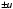of the value of the measurand is 0.58.

Quadrature: combining two or more quantities as the square root of the sum of the squares, i.e. $$\sqrt{(x_1)^2 + (x_2)^2 + ... + (x_N)^2 }$$.

Significant Figures: the number of digits in the expression of a number. The number of significant figures in the value of a measurand is defined by the uncertainty.

This Guide was written by David M. Harrison, Dept. of Physics, Univ. of Toronto, September 2013.

### Feedback Form

Do you have any comments or suggestions about the activities you did today? Are there any bugs, typos, or improvements you think would help future students? For specific problems please be sure to reference the exact section and paragraph or figure you are referring to.
Thanks!